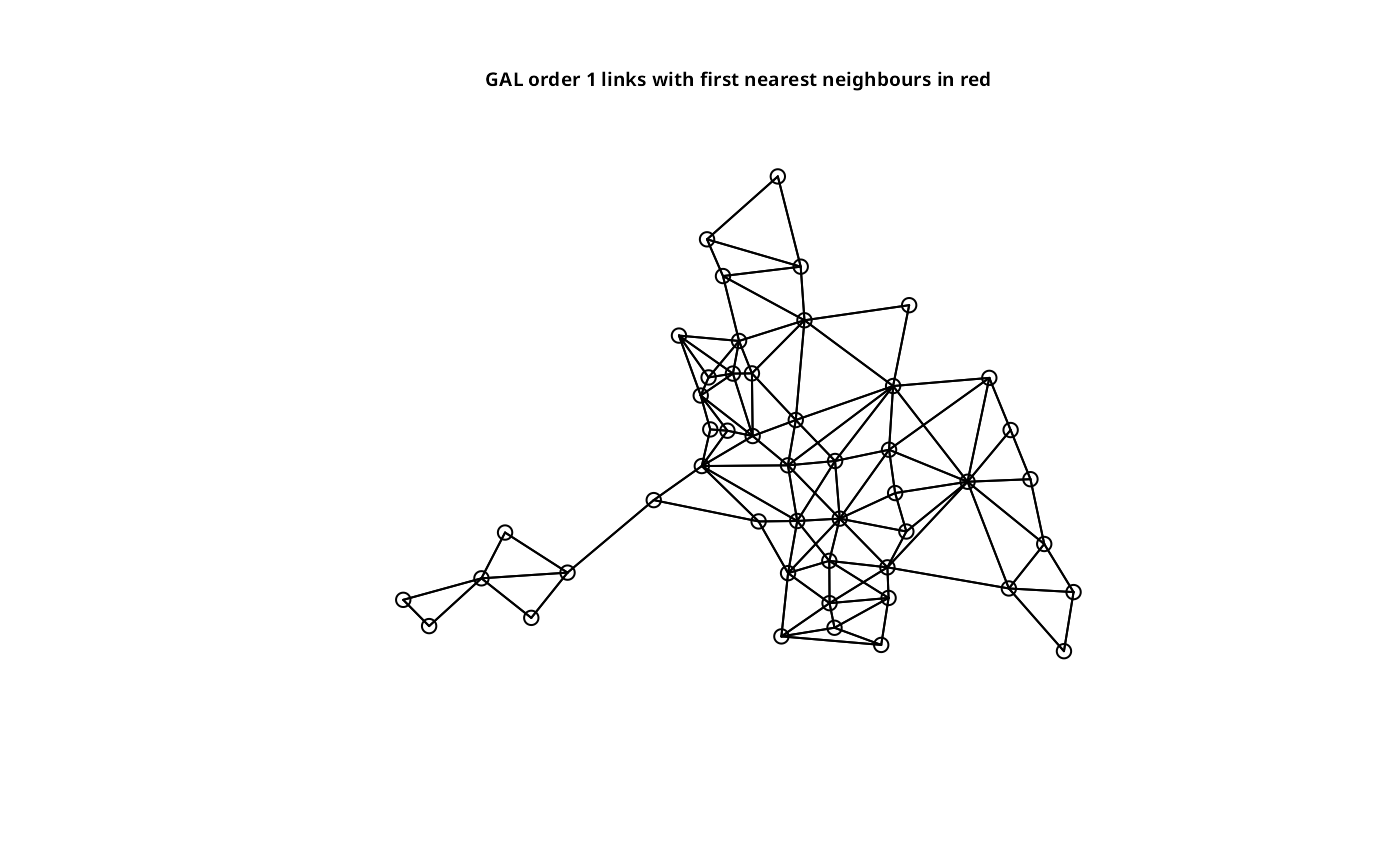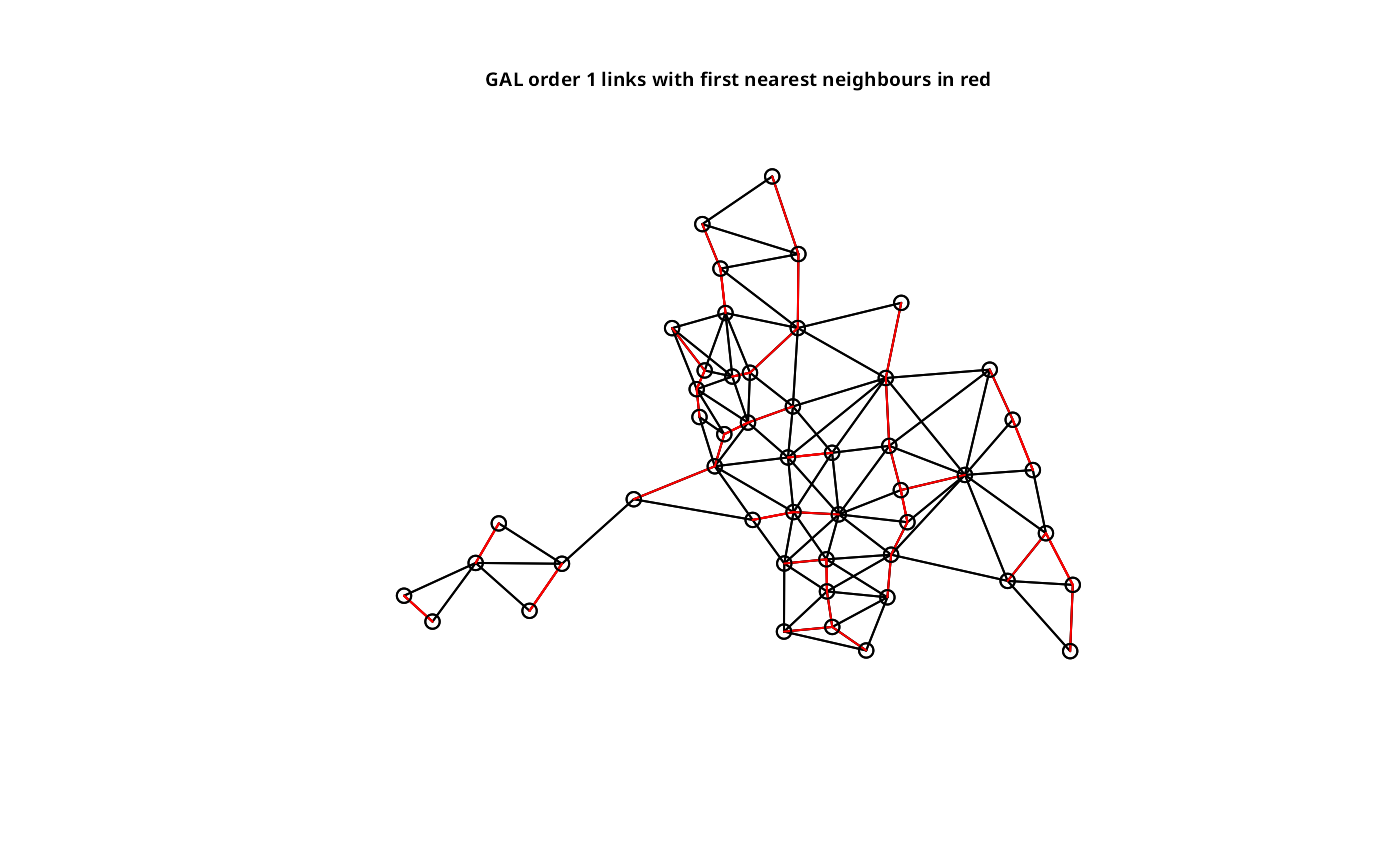A function to plot a neighbours list given point coordinates to represent the region in two dimensions; plot.listw is a wrapper that passes its neighbours component to plot.nb.

# S3 method for nb
plot(x, coords, col="black", points=TRUE, add=FALSE, arrows=FALSE,
length=0.1, xlim=NULL, ylim=NULL, ...)
# S3 method for listw
plot(x, coords, col="black", points=TRUE, add=FALSE, arrows=FALSE,
length=0.1, xlim=NULL, ylim=NULL, ...)

## Arguments

x

an object of class nb or (for plot.listw) class listw

coords

matrix of region point coordinates, a Spatial object (points or polygons), or an sfc object (points or polygons)

col

plotting colour

points

arrows

(logical) draw arrowheads for asymmetric neighbours

length

length in plot inches of arrow heads drawn for asymmetric neighbours lists

xlim, ylim

plot window bounds

...

further graphical parameters as in par(..)

## Author

Roger Bivand Roger.Bivand@nhh.no

summary.nb

## Examples

columbus <- st_read(system.file("shapes/columbus.shp", package="spData"), quiet=TRUE)